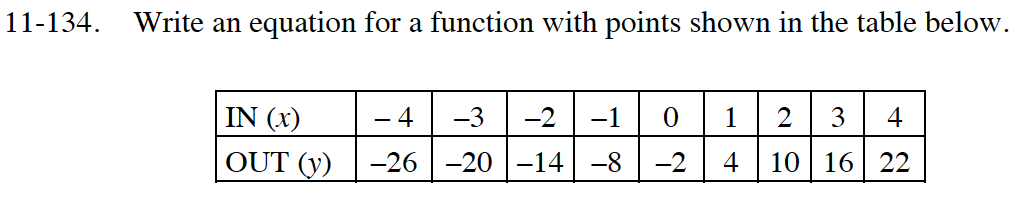Home > CC4 > Chapter 11 > Lesson 11.2.6 > Problem11-134

11-134.
1. Write an equation for a function with points shown in the table below. 11-134 HW eTool (Desmos). Homework Help ✎

 IN (x) −4 −3 −2 −1 0 1 2 3 4 OUT (y) −26 −20 −14 −8 −2 4 10 16 22Use the table to determine the slope and y-intercept of the line.

y = 6x − 2

Use the eTool below to write the equation represented by the table in the problem.
Click the link at the right to view full version of the eTool: Int1 11-134 HW eTool.